# Simulated annealing in Python¶

This small notebook implements, in Python 3, the simulated annealing algorithm for numerical optimization.

This notebook should be compatible with both Python versions, 2 and 3.

In :
from __future__ import print_function, division  # Python 2 compatibility if needed

In :
import numpy as np
import numpy.random as rn
import matplotlib.pyplot as plt  # to plot
import matplotlib as mpl

from scipy import optimize       # to compare

import seaborn as sns
sns.set(context="talk", style="darkgrid", palette="hls", font="sans-serif", font_scale=1.05)

FIGSIZE = (19, 8)  #: Figure size, in inches!
mpl.rcParams['figure.figsize'] = FIGSIZE


## Algorithm¶

The following pseudocode presents the simulated annealing heuristic.

• It starts from a state $s_0$ and continues to either a maximum of $k_{\max}$ steps or until a state with an energy of $e_{\min}$ or less is found.
• In the process, the call $\mathrm{neighbour}(s)$ should generate a randomly chosen neighbour of a given state $s$.
• The annealing schedule is defined by the call $\mathrm{temperature}(r)$, which should yield the temperature to use, given the fraction $r$ of the time budget that has been expended so far.

Simulated Annealing:

• Let $s$ = $s_0$
• For $k = 0$ through $k_{\max}$ (exclusive):
• $T := \mathrm{temperature}(k ∕ k_{\max})$
• Pick a random neighbour, $s_{\mathrm{new}} := \mathrm{neighbour}(s)$
• If $P(E(s), E(s_{\mathrm{new}}), T) \geq \mathrm{random}(0, 1)$:
• $s := s_{\mathrm{new}}$
• Output: the final state $s$

## Basic but generic Python code¶

In :
def annealing(random_start,
cost_function,
random_neighbour,
acceptance,
temperature,
maxsteps=1000,
debug=True):
""" Optimize the black-box function 'cost_function' with the simulated annealing algorithm."""
state = random_start()
cost = cost_function(state)
states, costs = [state], [cost]
for step in range(maxsteps):
fraction = step / float(maxsteps)
T = temperature(fraction)
new_state = random_neighbour(state, fraction)
new_cost = cost_function(new_state)
if debug: print("Step #{:>2}/{:>2} : T = {:>4.3g}, state = {:>4.3g}, cost = {:>4.3g}, new_state = {:>4.3g}, new_cost = {:>4.3g} ...".format(step, maxsteps, T, state, cost, new_state, new_cost))
if acceptance_probability(cost, new_cost, T) > rn.random():
state, cost = new_state, new_cost
states.append(state)
costs.append(cost)
# print("  ==> Accept it!")
# else:
#    print("  ==> Reject it...")
return state, cost_function(state), states, costs


## Basic example¶

We will use this to find the global minimum of the function $x \mapsto x^2$ on $[-10, 10]$.

In :
interval = (-10, 10)

def f(x):
""" Function to minimize."""
return x ** 2

def clip(x):
""" Force x to be in the interval."""
a, b = interval
return max(min(x, b), a)

In :
def random_start():
""" Random point in the interval."""
a, b = interval
return a + (b - a) * rn.random_sample()

In :
def cost_function(x):
""" Cost of x = f(x)."""
return f(x)

In :
def random_neighbour(x, fraction=1):
"""Move a little bit x, from the left or the right."""
amplitude = (max(interval) - min(interval)) * fraction / 10
delta = (-amplitude/2.) + amplitude * rn.random_sample()
return clip(x + delta)

In :
def acceptance_probability(cost, new_cost, temperature):
if new_cost < cost:
# print("    - Acceptance probabilty = 1 as new_cost = {} < cost = {}...".format(new_cost, cost))
return 1
else:
p = np.exp(- (new_cost - cost) / temperature)
# print("    - Acceptance probabilty = {:.3g}...".format(p))
return p

In :
def temperature(fraction):
""" Example of temperature dicreasing as the process goes on."""
return max(0.01, min(1, 1 - fraction))


Let's try!

In :
annealing(random_start, cost_function, random_neighbour, acceptance_probability, temperature, maxsteps=30, debug=True);

Step # 0/30 : T =    1, state = -7.45, cost = 55.5, new_state = -7.45, new_cost = 55.5 ...
Step # 1/30 : T = 0.967, state = -7.45, cost = 55.5, new_state = -7.44, new_cost = 55.4 ...
Step # 2/30 : T = 0.933, state = -7.44, cost = 55.4, new_state = -7.5, new_cost = 56.2 ...
Step # 3/30 : T =  0.9, state = -7.5, cost = 56.2, new_state = -7.59, new_cost = 57.6 ...
Step # 4/30 : T = 0.867, state = -7.59, cost = 57.6, new_state = -7.64, new_cost = 58.3 ...
Step # 5/30 : T = 0.833, state = -7.59, cost = 57.6, new_state = -7.51, new_cost = 56.4 ...
Step # 6/30 : T =  0.8, state = -7.51, cost = 56.4, new_state = -7.53, new_cost = 56.6 ...
Step # 7/30 : T = 0.767, state = -7.53, cost = 56.6, new_state = -7.58, new_cost = 57.5 ...
Step # 8/30 : T = 0.733, state = -7.53, cost = 56.6, new_state = -7.6, new_cost = 57.8 ...
Step # 9/30 : T =  0.7, state = -7.53, cost = 56.6, new_state = -7.51, new_cost = 56.4 ...
Step #10/30 : T = 0.667, state = -7.51, cost = 56.4, new_state = -7.24, new_cost = 52.4 ...
Step #11/30 : T = 0.633, state = -7.24, cost = 52.4, new_state = -6.98, new_cost = 48.7 ...
Step #12/30 : T =  0.6, state = -6.98, cost = 48.7, new_state = -6.6, new_cost = 43.5 ...
Step #13/30 : T = 0.567, state = -6.6, cost = 43.5, new_state = -6.69, new_cost = 44.8 ...
Step #14/30 : T = 0.533, state = -6.6, cost = 43.5, new_state = -6.84, new_cost = 46.8 ...
Step #15/30 : T =  0.5, state = -6.6, cost = 43.5, new_state = -6.45, new_cost = 41.6 ...
Step #16/30 : T = 0.467, state = -6.45, cost = 41.6, new_state = -6.24, new_cost = 38.9 ...
Step #17/30 : T = 0.433, state = -6.24, cost = 38.9, new_state = -6.52, new_cost = 42.5 ...
Step #18/30 : T =  0.4, state = -6.24, cost = 38.9, new_state = -5.92, new_cost = 35.1 ...
Step #19/30 : T = 0.367, state = -5.92, cost = 35.1, new_state = -6.35, new_cost = 40.4 ...
Step #20/30 : T = 0.333, state = -5.92, cost = 35.1, new_state = -5.98, new_cost = 35.8 ...
Step #21/30 : T =  0.3, state = -5.92, cost = 35.1, new_state = -5.35, new_cost = 28.6 ...
Step #22/30 : T = 0.267, state = -5.35, cost = 28.6, new_state = -4.67, new_cost = 21.8 ...
Step #23/30 : T = 0.233, state = -4.67, cost = 21.8, new_state = -4.44, new_cost = 19.7 ...
Step #24/30 : T =  0.2, state = -4.44, cost = 19.7, new_state = -4.59, new_cost = 21.1 ...
Step #25/30 : T = 0.167, state = -4.44, cost = 19.7, new_state = -4.04, new_cost = 16.3 ...
Step #26/30 : T = 0.133, state = -4.04, cost = 16.3, new_state = -4.77, new_cost = 22.8 ...
Step #27/30 : T =  0.1, state = -4.04, cost = 16.3, new_state = -4.7, new_cost = 22.1 ...
Step #28/30 : T = 0.0667, state = -4.04, cost = 16.3, new_state = -3.44, new_cost = 11.8 ...
Step #29/30 : T = 0.0333, state = -3.44, cost = 11.8, new_state = -2.6, new_cost = 6.78 ...


Now with more steps:

In :
state, c, states, costs = annealing(random_start, cost_function, random_neighbour, acceptance_probability, temperature, maxsteps=1000, debug=False)

state
c

Out:
0.08501260465044064
Out:
0.007227142949452121

## Visualizing the steps¶

In :
def see_annealing(states, costs):
plt.figure()
plt.suptitle("Evolution of states and costs of the simulated annealing")
plt.subplot(121)
plt.plot(states, 'r')
plt.title("States")
plt.subplot(122)
plt.plot(costs, 'b')
plt.title("Costs")
plt.show()

In :
see_annealing(states, costs)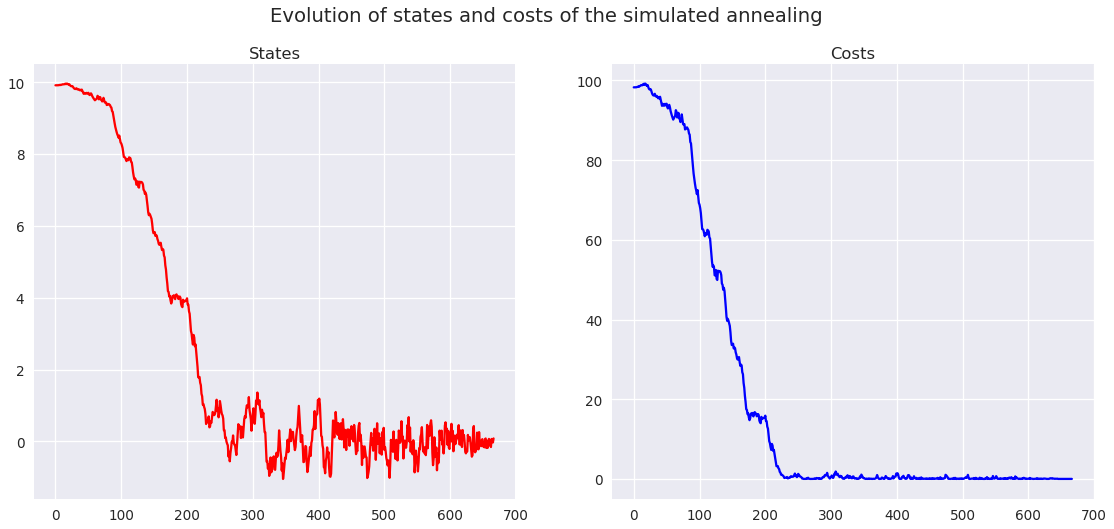## More visualizations¶

In :
def visualize_annealing(cost_function):
state, c, states, costs = annealing(random_start, cost_function, random_neighbour, acceptance_probability, temperature, maxsteps=1000, debug=False)
see_annealing(states, costs)
return state, c

In :
visualize_annealing(lambda x: x**3)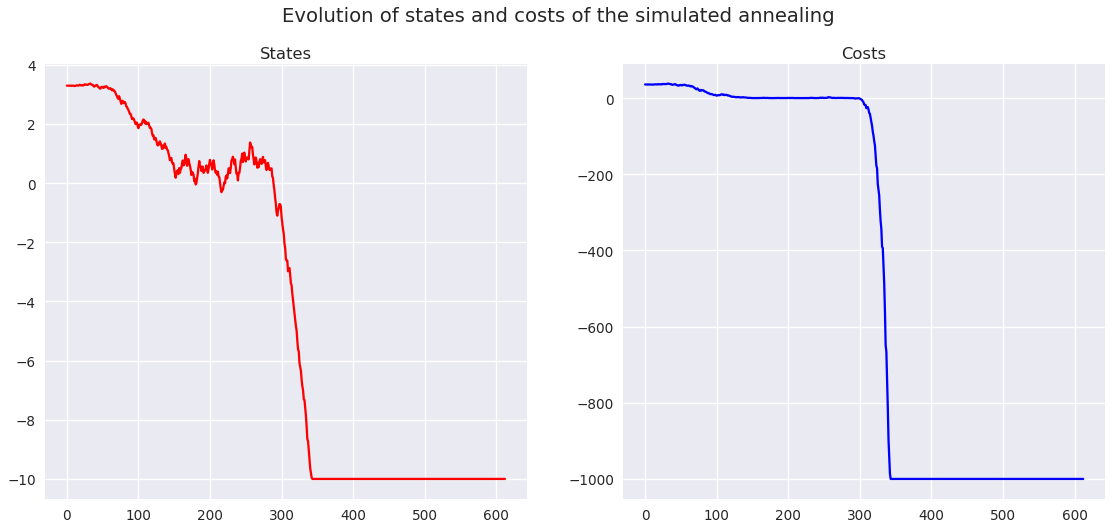Out:
(-10, -1000)
In :
visualize_annealing(lambda x: x**2)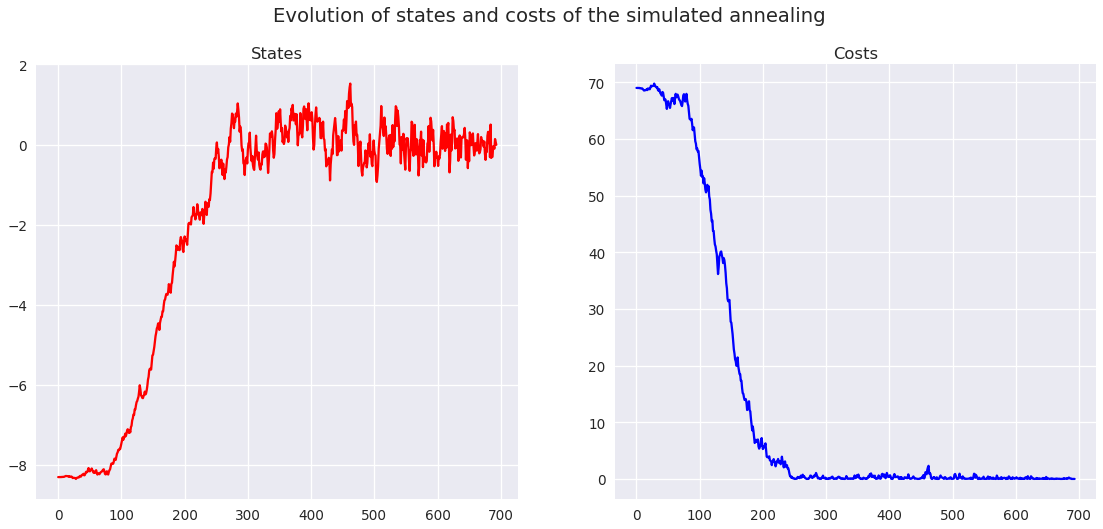Out:
(-0.007545319790391913, 5.6931850739279866e-05)
In :
visualize_annealing(np.abs)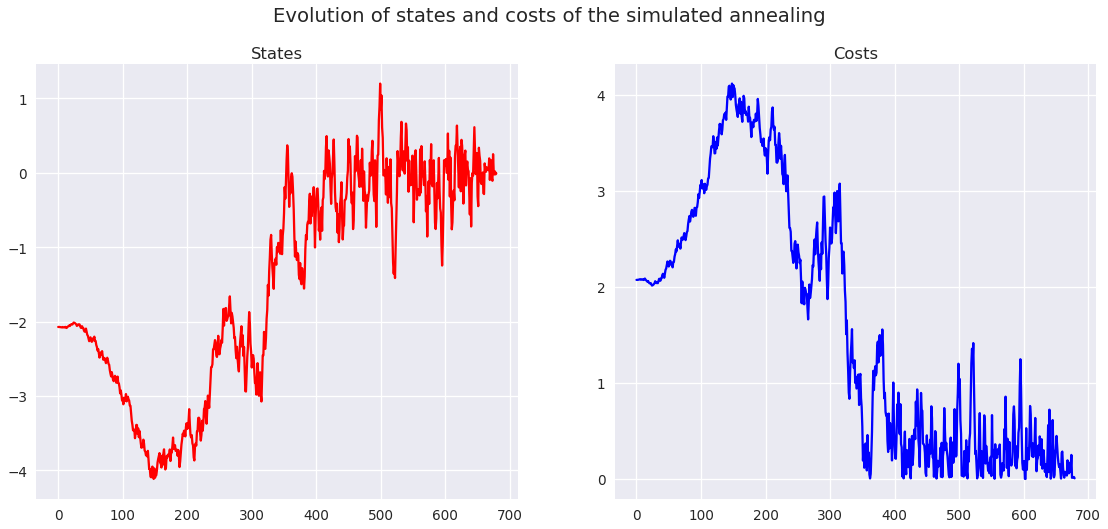Out:
(-0.007160473894364527, 0.0071604738943645274)
In :
visualize_annealing(np.cos)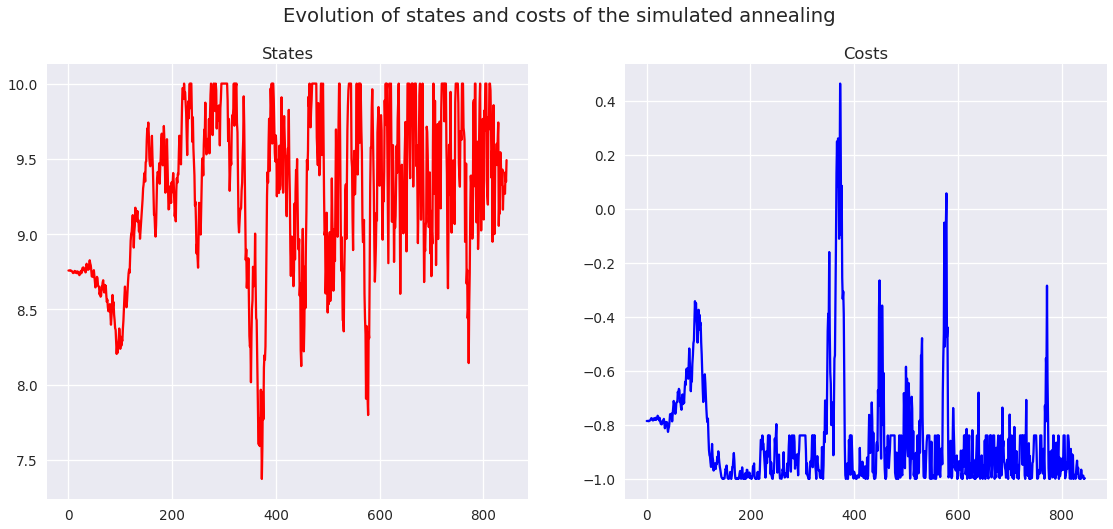Out:
(9.491236787419837, -0.9977924248899227)
In :
visualize_annealing(lambda x: np.sin(x) + np.cos(x))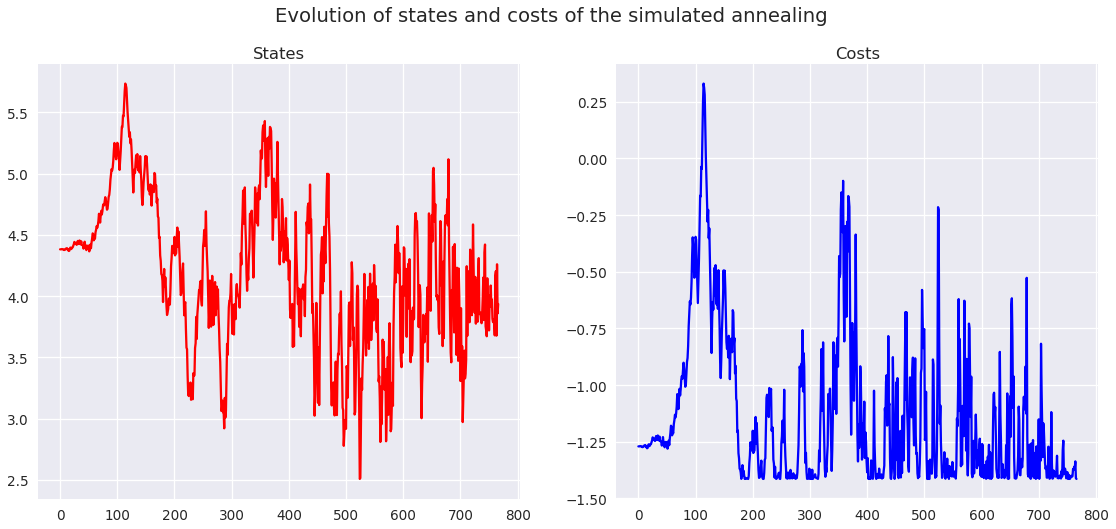Out:
(3.935615512590335, -1.4141609642965898)

In all these examples, the simulated annealing converges to a global minimum. It can be non-unique, but it is found.

That's it for today, folks!

More notebooks can be found on my GitHub page.